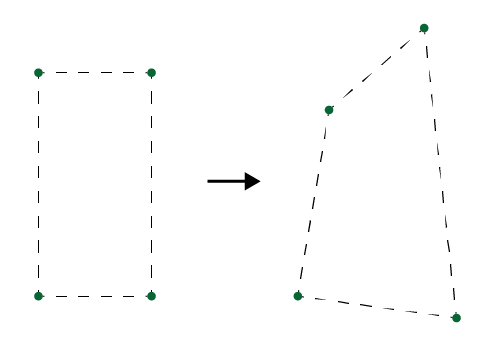# How to invert an 8x8 matrix or calculate 8DOF homography

Hi, I’m trying to write my own distortion tool. I have 2 sets of corners and want to solve for the transformation matrix between them. I found I can do that by solving the system below for H but it means I need to invert an 8x8 matrix. How do I do that? or otherwise how do I find the transformation matrix from the points?

Edit: I’m trying to find the 4x4 matrix H=[h11, …, h32] that transformed 4 given pairs of 2d points. Once I have it I can use it to transform all of a mesh’s vertices and get a distorted mesh (I’ll do it by extending BaseMeshEffects). So I need to invert the large matrix on the left & Left-multiply the inverse by the vector on the right to get H’s coefficients.Well I’m not sure what you actually want to do here. However to invert a 8x8 matrix (or any sized matrix) you can use my GaussMatrixF which is just an arbitrary sized matrix which can be solved by Gaussian elimination. It’s meant to be setup as an augmented matrix and it will solve the first square part of the matrix into an identity matrix. So to solve an 8x8 matrix you would need to create a 8x16 matrix (8 rows, 16 columns) and place you original matrix in the left half. Fill the right half with the 8x8 identity matrix and call Solve. It’s explained here.

Something like this:

``````var gm = GaussMatrixF(16, 8);
gm.rows.cols = x1;
gm.rows.cols = y1;
// [ ... ]
// fill your whole 8x8 matrix
// [ ... ]

// initialize second half with identity matrix
for(int r = 0; r < 8 r++)
{
for(int c = 0; c < 8 c++)
{
if (r == c)
gm.rows[r].cols[8+c] = 1;
else
gm.rows[r].cols[8+c] = 0;
}
}

if (gm.Solve())
{
// access inverted matrix by reading back columns index 8 to 15
}
``````